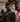# Crafting Interpreters in OCaml - Lexing

May 15, 2019

An interpreter is a program that takes some input code and immediately executes it.

There are multiple steps to an interpreter that Bob Nystrom covers in detail on his website. For the first pass, we’ll be building a tree-walking interpreter. This shrinks the problem domain a bit. In a nutshell, we’ll have to solve the following problems.

Step one is lexical analysis - taking in some stream of text (the code input) and breaking it into chunks or lexemes. For example, if I were to type

``const x = 2;``

we have 5 lexemes - the keyword `const`, the variable `x`, the equals sign, the integer `2`, and the semicolon. However, in order to maintain the context or type of lexeme, we’re going to have to tag them with a type. You can think of each chunk broken into a token, which might look like the following

``````  const token1 = {
lexeme: "=",
type: "EQUALS"
}

const token2 = {
lexeme: "2",
type: "NUMBER"
}``````

in OCaml we’d use the built in type system and end up with something more like

``````type token_type = EQUALS | NUMBER
type token = {
lexeme: string;
type: token_type;
}

let token1 = {
lexeme = "=";
type: EQUALS
}

let token1 = {
lexeme = "2";
type: NUMBER
}``````

Step two is parsing - taking our list of tokens and building an abstract syntax tree. This tree representation lets us build out a data structure that can throw errors when leaf nodes are invalid, recurse and find subexpressions, etc.

If you were to imagine the following

``  (1 + 2) / 3``

This might be represented as nodes of

``````  type fn = Add | Subtract | Multiply | Divide
let tree =
| Literal of int
| Node of fn * tree * tree

Node(
Divide,